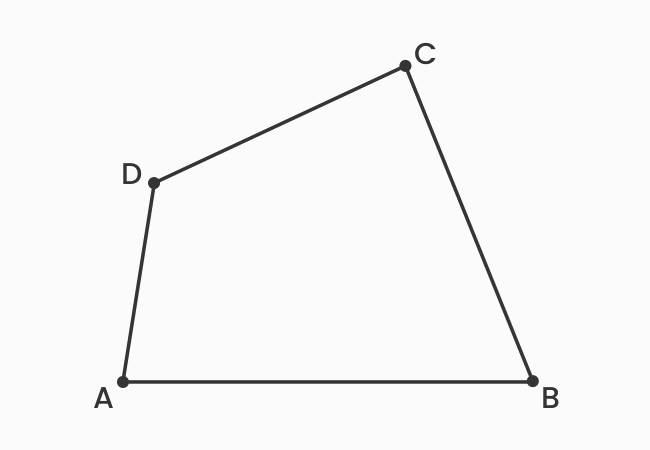The sides, which have a common vertex in a quadrilateral are called the adjacent sides of a quadrilateral.

## Introduction

There are four line-segments in a quadrilateral. The endpoint of one line segment is connected to the endpoint of another line segment to form a closed geometric shape. Thus, every two sides are connected at an intersecting point, called a vertex, and the two sides are called the adjacent sides of a quadrilateral.The adjacent sides are also called the consecutive sides of a quadrilateral. There are four pairs of consecutive sides in a quadrilateral. Now, let’s identify four pairs of adjacent sides in a quadrilateral.

1. The sides $\small \overline{AB}$ and $\small \overline{BC}$ have a common intersecting point at point $\small B$. So, the sides $\small \overline{AB}$ and $\small \overline{BC}$ are called the adjacent sides of quadrilateral.
2. The sides $\small \overline{BC}$ and $\small \overline{CD}$ have a vertex at point $\small C$. So, the sides $\small \overline{BC}$ and $\small \overline{CD}$ are called the consecutive sides of quadrilateral.
3. The sides $\small \overline{CD}$ and $\small \overline{DA}$ have a common meeting point at point $\small D$. So, the sides $\small \overline{CD}$ and $\small \overline{DA}$ are called the adjacent sides of quadrilateral.
4. The sides $\small \overline{DA}$ and $\small \overline{AB}$ have a vertex $\small D$ commonly. Therefore, the sides $\small \overline{DA}$ and $\small \overline{AB}$ are called the consecutive sides of quadrilateral.
Latest Math Topics
Jun 26, 2023
Jun 23, 2023

Latest Math Problems
Jul 01, 2023
Jun 25, 2023
###### Math Questions

The math problems with solutions to learn how to solve a problem.

Learn solutions

Practice now

###### Math Videos

The math videos tutorials with visual graphics to learn every concept.

Watch now

###### Subscribe us

Get the latest math updates from the Math Doubts by subscribing us.